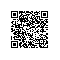# 《C++覆辙录》——1.8：未能区分可访问性和可见性

### 1.8：未能区分可访问性和可见性

C++语言压根儿没有实现什么数据隐藏，它实现了的是访问层级。在class中具有protectedprivate访问层级并非不可见，只是不能访问罢了。如同一切可见而不可及的事物一样（经理的形象跃入脑海），他们总是惹出各种麻烦。

class C {
public:
C( int val ) : a_( val ),
b_( a_ ) // 新添加的代码
{}
int get_a() const { return a_; }
int get_b() const { return b_; } // 新添加的代码
private:
int b_; // 新添加的代码
int a_;
};

class C;

template
class Cbase{
// ...
};
typedef Cbase C;

#include "c.h"
// ...
class C; // 错误！C现在不再是class的名字，而是typedef。



class C {
public:
C( int val );
～C();
int get_a() const;
int get_b() const;
private:
Cimpl *impl_;
};

class Cimpl {
public:
Cimpl( int val ) : a_( val ), b_( a_ ) {}
～Cimpl() {}
int get_a() const { return a_; }
int get_b() const { return b_; }
private:
int a_;
int b_;
};
C::C( int val )
: impl_( new Cimpl( val ) ) {}
C::～C()
{ delete impl_; }
int C::get_a() const
{ return impl_->get_a(); }
int C::get_b() const
{ return impl_->get_b(); }

class B {
public:
void g();
private:
virtual void f(); // 新添加的代码
};
class D : public B {
public:
void f();
private:
double g; // 新添加的代码
};
class B这个基类中添加了一个私有访问的虚函数，导致了原先派生类中的非虚函数变成了虚函数，添加在class D中的私有访问的数据成员则遮掩了B中的一个函数。这就是为什么继承常常被视为“白盒”复用34，因为对class的任何修改都在非常基本的层面同时影响着基类和派生类的语义。使用钉钉扫一扫加入圈子
+ 订阅